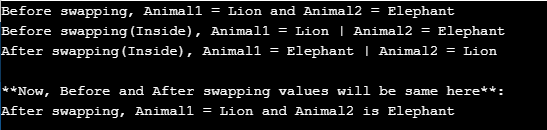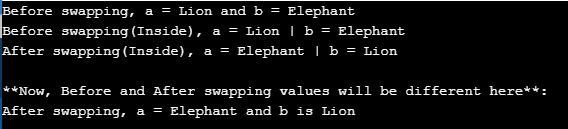# Pass By Value and Pass By Reference in Java

## Pass By Value and Pass By Reference in Java

In this article, I am going to discuss Pass By Value and Pass By Reference in Java with Examples. Please read our previous article, where we discussed Java User Input and Output with examples. At the end of this article, you will understand Pass By Value and Pass By Reference in the Java application.

One of the biggest confusion in Java programming language is whether java is Pass by Value or Pass by Reference. Many programming languages allow passing parameters by reference or by value. In Java, we can only pass parameters by value.  First of all, we should understand what is meant by pass by value and pass by reference.

##### Pass By Value in Java:

Actual parameter expressions that are passed to a method are evaluated and value is derived. Then this value is stored in a small portion of the memory and a copy of each value is passed to the parameters of the called method which can then be used. This mechanism is called pass-by-value and Java uses it.

In the call by value, the modification done to the values passed does not reflect in the caller’s scope because when these values are used inside the method, we are actually using the copy of these values, not the original argument values which are unaffected by the operation inside the method.

##### Example to Understand Pass By Value Mechanism in Java:
```package Demo;
public class PassByValueDemo
{
public static void main (String[]args)
{
String animal1 = "Lion";
String animal2 = "Elephant";
System.out.println ("Before swapping, Animal1 = " + animal1 + " and Animal2 = " + animal2);

// Invoke the swap method
Swap(animal1, animal2);
System.out.println("\n**Now, Before and After swapping values will be same here**:");
System.out.println ("After swapping, Animal1 = " + animal1 +" and Animal2 is " + animal2);
}

public static void Swap(String animal1, String animal2)
{
System.out.println ("Before swapping(Inside), Animal1 = " + animal1 +" | Animal2 = " + animal2);
// Swap animal1 with animal2
String tempAnimal = animal1;
animal1 = animal2;
animal2 = tempAnimal;
System.out.println ("After swapping(Inside), Animal1 = " + animal1 +" | Animal2 = " + animal2);
}
}
```
###### Output:##### Pass By Reference in Java:

Call by reference method copies the address of an argument into the formal parameter. In this method, the address is used to access the actual argument used in the method call. When we pass a variable of class type as an argument to a method, actually, a copy of a reference (address) to an object is passed by value to the method, not a copy of the object itself because Java does not copy the object into the memory.

Java uses only call by value while passing reference variables as well. Actually, it copies the reference of the object into the memory and passes the copy to the parameters of the called method. If we change the reference of the object then the original reference does not get changed because this reference is a copy.

##### Example to Understand Pass By Reference Mechanism in Java:
```package Demo;
public class PassByreferenceDemo
{
public static void main (String[]args)
{
Animal a = new Animal("Lion");
Animal b = new Animal("Elephant");
System.out.println ("Before swapping, a = " + a.a + " and b = " + b.a);

// Invoke the swap method
swapFunction (a, b);
System.out.println("\n**Now, Before and After swapping values will be different here**:");
System.out.println ("After swapping, a = " + a.a + " and b is " + b.a);
}

public static void swapFunction (Animal a, Animal b)
{
System.out.println ("Before swapping(Inside), a = " + a.a + " | b = " +b.a);
// Swap n1 with n2
Animal c = new Animal (a.a);
a.a = b.a;
b.a = c.a;
System.out.println ("After swapping(Inside), a = " + a.a + " | b = " +b.a);
}
}

class Animal
{
public String a;
public Animal (String a)
{
this.a = a;
}
}```
###### Output:NOTE: Java passes the arguments both primitives and the copy of object reference by value. Java never passes the object itself.

Thus, by this tutorial, it is clear that Java uses the pass-by-value. There is no pass-by-reference in Java. “The reference is copied as a value” to a new variable and it is given as a formal parameter to the called method.

Just remember that variables are references and their copies are passed to the methods, so java is always pass-by-value.

##### Difference Between Pass By Value and Pass By Reference
 Pass By Value Pass By Reference A way of calling a function in which the address of the actual arguments is copied to the formal parameters. A method of passing the argument to a function by copying the reference as a value of an argument into the formal parameter. The programmer passes the address of the actual arguments to formal parameters The programmer passes the references of the actual arguments to the formal parameters. Memory is allocated for both actual arguments and formal parameters. Memory is allocated only for actual arguments and formal parameters share that memory.